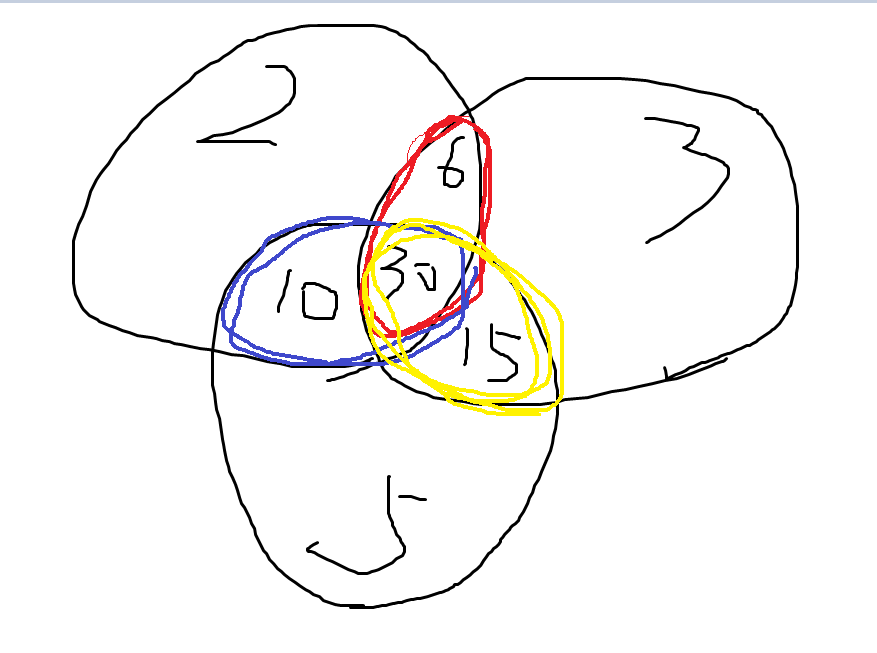I - Co-prime

Given a number N, you are asked to count the number of integers between A and B inclusive which are relatively prime to N.
Two integers are said to be co-prime or relatively prime if they have no common positive divisors other than 1 or, equivalently, if their greatest common divisor is 1. The number 1 is relatively prime to every integer.

Input

The first line on input contains T (0 < T <= 100) the number of test cases, each of the next T lines contains three integers A, B, N where (1 <= A <= B <= 10^15) and (1 <=N <= 10^9).


Output

For each test case, print the number of integers between A and B inclusive which are relatively prime to N. Follow the output format below.


Sample Input

2
1 10 2
3 15 5


Sample Output

Case #1: 5
Case #2: 10


Hint

In the first test case, the five integers in range [1,10] which are relatively prime to 2 are {1,3,5,7,9}.


## 原理## 分析题解

ok，讲完了容斥的原理，接下来就能做题了，题目要求区间段内与k互质的个数，就是求区间段内与k不互质的个数，然后再用区间长度减去这个数就行了，求[l,r]中与k不互质的个数，就是求[1,r]-[1,l-1]（一定注意是l-1，因为包括l），理清了思路，就可以敲代码了

## 分解质因子的代码

for(int i=2;i*i<=k;i++){
if(k%i==0){
p[++tail]=i;  //p就是储存质因子的数组
while(k%i==0) k/=i;  //把k中所有i的质因子全部除去
}
}
if(k>1) p[++tail]=k;  //最后如果大于一，则最后一个数一定是质因子，这一步可能有一点难理解，可以多想想


## 实现容斥定理的代码

long long fun(long long x){
long long res=0;  //res储存的是1-x中与K不互质的数量
for(int i=1;i<(1<<tail);i++){  //这里的1<<tail是指2的tail次方，表示tail个质因子有多少种组合情况
long long cur=1,cnt=0;  //cur表示在当前选中的质因子中的乘积，cnt表示当前选中的数量是奇数还是偶数
for(int j=0;j<tail;j++){  //这个循环是枚举tail的二进制形式
if((i>>j)&1){  //这个是判断i的第j位是不是1，如果是则表示选中第j个数
cnt++;  //表示选中了几个数，每选中一个就加一
cur*=p[j+1];  //选中第j个数就用cur乘以第j个质因子数，注意质因子数组是从1开始的，所以要加一
}
}
if(cnt&1) res+=x/cur;  //如果cnt是偶数就相加
else res-=x/cur;  //奇数就相减
}
return x-res;  //res储存的是1-x中与K不互质的数量，所以要用x-res得到互质的数量
}


#include<iostream>
#include<stdio.h>
#include<algorithm>
using namespace std;
int p;
int tail;
long long fun(long long x){
long long res=0;
for(int i=1;i<(1<<tail);i++){
long long cur=1,cnt=0;
for(int j=0;j<tail;j++){
if((i>>j)&1){
cnt++;
cur*=p[j+1];
}
}
if(cnt&1) res+=x/cur;
else res-=x/cur;
}
return x-res;
}
int main()
{
int t,times=0;
cin>>t;
while(t--){
tail=0;
long long l,r,k;
cin>>l>>r>>k;
for(int i=2;i*i<=k;i++){
if(k%i==0){
p[++tail]=i;
while(k%i==0) k/=i;
}
}
if(k>1) p[++tail]=k;
long long ans=fun(r)-fun(l-1);
printf("Case #%d: %lld\n",++times,ans);
}
}


••